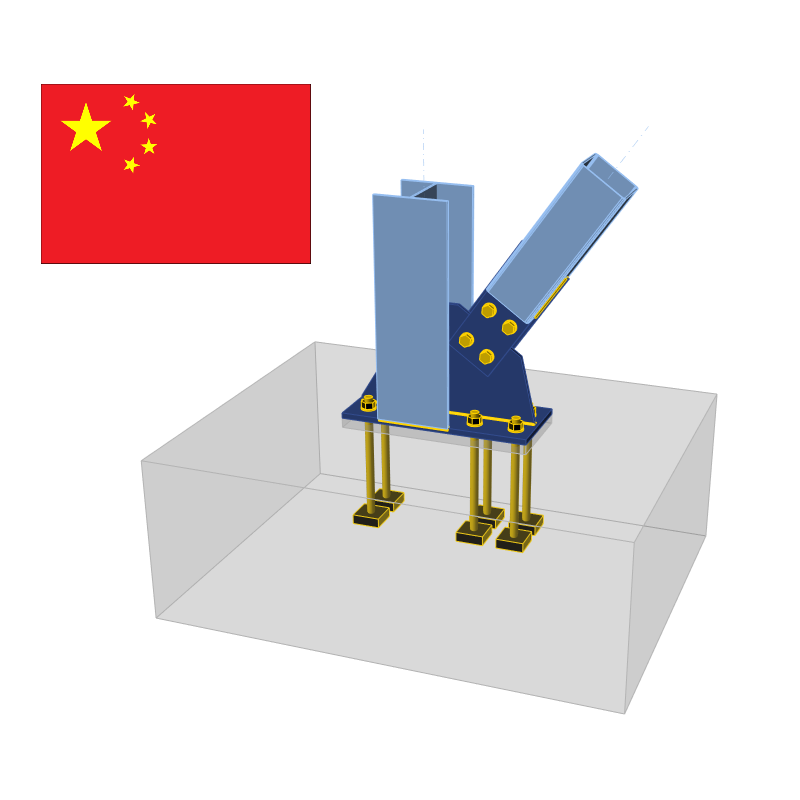### Choose language# Check of components according to GB (Chinese standards)

$$CBFEM method combines advantages of the general Finite Element Method (FEM) and standard Component Method (CM). The stresses and internal forces calculated on the accurate CBFEM model are used in checks of all components – Bolts, preloaded bolts and welds are checked according to GB 50017 – 2017. The plates are checked by finite element analysis. The checks of anchorage have not yet been implemented in the current version. Design check of plates according to Chinese Standard The resulting equivalent stress (HMH, von Mises) and plastic strain are calculated on plates. When the design yield strength, f (GB 50017, Table 4.4.1–4.4.3), on the bilinear material diagram is reached, the check of the equivalent plastic strain is performed. The limit value of 5 % is suggested in Eurocode (EN 1993-1-5 App. C, Par. C8, Note 1). This value can be modified in Code setup but verification studies were made for this recommended value.Plate element is divided into 5 layers and elastic/plastic behaviour is investigated in each of them. The program shows the worst result of all of them.Stress may be a little bit higher than design yield strength. The reason is the slight inclination of the plastic branch of the stress-strain diagram, which is used in the analysis to improve the stability of the calculation. Check of bolts and preloaded bolts according to Chinese standard BoltsBolts are checked according to GB 50017, Cl. 11.4. The tensile and shear force in each bolt is determined by finite element analysis. Prying forces are determined by finite element analysis and taken into account. Each shear plane is checked individually. Plate in bearing is checked against the sum of shear forces at nearby planes.Design tensile and shear strengths of a bolt; fub[MPa] – ultimate strength of a bolt; derived from Table 4.4.6 $$f_{ub}$$ [MPa]$$f_t^b$$$$f_v^b$$ $$f_{ub} \le 400$$$$0.425 \cdot f_{ub}$$$$0.35 \cdot f_{ub}$$ \(400